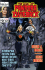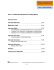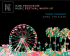# View a sample Math in Focus lesson

## Transcription

View a sample Math in Focus lesson
```MATH • Lesson 57
Lesson 57
Simplifying Expressions
Objectives
• Identify, write, simplify, and evaluate
expressions with a variable.
Books & Materials
• Math in Focus 5A
• Workbook 5A
• 10 craft sticks (Optional)
CCSS K–12.MP2, 4, 6,
and 7
Assignments ❑❑ Complete Warm-up.
❑❑ Read and complete pp. 219–223 and 225,
Math in Focus 5A.
❑❑ Complete pp. 183–186, Workbook 5A.
❑❑ Complete Math Checkpoint.
Warm-up.
Write these addition expressions as multiplication expressions and solve.
1. 5 + 5 + 5 + 5 + 5 + 5 + 5
2. 6 + 6 + 6
3. 9 + 9 + 9 + 9
4. 2 + 2 + 2 + 2 + 2
5. 8 + 8 + 8 + 8 + 8 + 8
6. 7 + 7 + 7
Instruction
Read Learn on pp. 219–220 in Math in Focus. As you saw in the Warm-up
activity, multiplication is a simpler way to write repeated addition. You can
simplify addition expressions with the same variable by writing a single
multiplication expression. For example, a + a + a is simplified by writing the
algebraic expression 3a. Complete Guided Practice on p. 220.
Read Learn in the middle of p. 220. You can only simplify variables with like
terms. For example, you can simplify x + 4x by writing 5x, but you cannot
simplify x + 4y because the variables are not alike; they are not like terms.
Complete Guided Practice on p. 220.
Read Learn on p. 221. If you subtract y − y, the answer is 0. Complete
Guided Practice on p. 221.
Read Learn on p. 221. Like terms can be subtracted from one another. For
example, in 4y – y, think 4 groups of y minus 1 group of y. Complete Guided
Practice on p. 222.
05M-LMA
Calvert Education Services • Grade 5 MATH Lesson 57﻿
129
Lesson 57 • MATH
Read Learn on p. 222. When you see an algebraic expression that has
multiple operations, remember the order of operations when you simplify
the expression. Complete Guided Practice on p. 222.
Read Learn on p. 223. When you simplify an expression, make sure to
combine like terms. It may be helpful to change the order of the problem
before you combine the terms. Complete Guided Practice on p. 223.
Instructional Video: Simplifying Algebraic Expressions
To the Learning Guide
When your student simplifies an expression, he may need to change the order of
the terms in the expression. For example, in a problem like 4x + 7 + x + 3, he should
group like terms. 4x + x + 7 + 3 is the same as 5x + 10.
The Commutative Property of Addition will show your student that he can change
the order of the addends and still get the same answer.
The optional Hands-On Activity on pp. 224–225 provides your student with an
opportunity to practice simplifying algebraic expressions. If he struggles with the
concept of simplifying expressions, continue working with the craft sticks to model
Watch For These Common Errors
!!
Some students may want to simplify an answer like 5x + 7 further.
Explain this expression cannot be further simplified because it does
not contain like terms.
Practice
Complete Let’s Practice on p. 225 in Math in Focus. Then complete
pp. 183–186 in Workbook.
Wrap-up
Today you learned how to simplify algebraic expressions.
m + m + m = 3 × m = 3m 3p – p = 2p 5k + 4 + k – 1 = 6k + 3
Complete Math Checkpoint
05M-LMA
130
Lesson 57 ﻿
Grade 5 MATH • Calvert Education Services
MATH • Lesson 58
Lesson 58
Working with Algebraic
Expressions, Equations,
and Inequalities
Objectives
• Write and evaluate inequalities.
• Use the properties of equality to
solve equations.
Books & Materials
• Math in Focus 5A
• Workbook 5A
• pan balance
• marbles, connecting cubes, pennies,
or other small items that have
nearly-identical masses (Optional)
CCSS 5.OA.1
K–12.MP1 and 2
Assignments ❑❑ Complete Warm-up.
❑❑ Read and complete pp. 226–231 Math in Focus 5A.
❑❑ Complete Math Checkpoint.
Warm-up
1. Place two pennies on both sides of the pan balance. What happens?
2. Now put four pennies on both sides of the pan balance. What happens?
3. Now, take one penny away from each side. Explain what happens.
Instruction
Read Learn on p. 226 in Math in Focus. As you read, watch how the terms
equation and inequality are used. An equation is a mathematical statement
with equal values on both sides. For example, in 2 + 2 = 4, the value on each
side of the equal sign equals 4.
An inequality is a statement that is not equal; each side has a different value.
For example, in 2 + 3 > 4, one side of the inequality has a value of 5, and the
other side has a value of 4.
Think about the pan balance when deciding if you have an equation or
inequality. If the values are the same on either side, it is an equation. If they
are not the same, it is an inequality. Complete Guided Practice at the top
of p. 227.
Then read Learn on p. 227. You can compare algebraic expressions when you
are given a value for the variable. Complete Guided Practice on p. 228.
Read Learn on pp. 228–231. Study the pictures of the balances carefully.
Then complete Guided Practice on p. 231.
05M-LMA
Calvert Education Services • Grade 5 MATH Lesson 58﻿
131
Lesson 58 • MATH
Instructional Video: One-Step Equations with Addition and Subtraction
Instructional Video: One-Step Equations with Multiplication and Division
Instructional Video: Inequalities
To the Learning Guide
The pan balance is a helpful tool for demonstrating balanced equations. It is a
concrete way to help your student understand this foundational algebraic concept.
If your student would like more practice, with equations, try this activity. Have him
cover his eyes or turn around so he cannot see the pan balance. On one pan place
5 pennies and then cover them with a small piece of tissue. Next to the covered
pennies place 2 pennies that are visible. On the other pan put 7 pennies. Ask your
student to open his eyes or turn around.
First, make sure he notes the balance is level. Tell him that under the tissue is a
certain number of pennies. Point out that he does not know the number of pennies
and have him assign a variable to this unknown value. Next, ask him to write an
equation for what he sees (x + 2 = 7) and to solve the equation. He can lift the tissue
to check his answer.
Finally, have your student take 1 penny away from each side and to write this
equation. (5 + 2 – 1 = 7 – 1) Continue the activity with different numbers of pennies
until your student feels confident about these concepts.
Practice
Complete Guided Practice on p. 228 in Math in Focus.
Wrap-up
Today you worked with a pan balance to show algebraic expressions as
inequalities and equations. When you know the value of the variable, you
can compare expressions.
p=4
4p = 16
2+p>5
Complete Math Checkpoint
05M-LMA
132
Lesson 58 ﻿
Grade 5 MATH • Calvert Education Services
MATH • Lesson 59
Lesson 59
Solving Equations
Objectives
• Use the properties of equality to
solve equations.
Books & Materials
• Math in Focus 5A
• Workbook 5A
• counters (Optional)
• connecting cubes (Optional)
• small paper cups (Optional)
• ruler (Optional)
CCSS 5.OA.1
K–12.MP1 and 2
Assignments ❑❑ Complete Warm-up.
❑❑ Read and complete pp. 232–235, Math in Focus 5A.
❑❑ Complete pp. 187–188, Workbook 5A.
❑❑ Complete Math Checkpoint.
Warm-up
Complete each number statement with >, <, or =.
1. x = 6 5x + 1
15 + x
2. y = 3 7y – 1
25 – y
3. a = 5 4 + 2a
2a + 4
Instruction
Read Learn on pp. 232–233. When you have variables on both sides of the
equal sign, isolate the variable, or rename the equation until the variable is
on one side of the equation and the numerical value is on the other. When
you find an answer, check your solution by substituting the number for the
variable in the original equation. When you simplify the expression, you
should have a true number statement.
Complete Guided Practice on p. 234.
Instructional Video: Solving Equations with Variables on Both Sides
To the Learning Guide
Your student may still need help visualizing how to solve the equation. Show him
how to model the problem by using connecting cubes to represent the variables,
empty paper cups to represent numbers being subtracted, and counters to represent
numbers being added. Set down a ruler to separate the 2 sides of the equation.
The equation 3p + 4 = 5p – 6 would be modeled as follows:
05M-LMA
Calvert Education Services • Grade 5 MATH Lesson 59﻿
133
Lesson 59 • MATH
counters
connecting cubes
ruler
cups
First your student must place 1 counter in each cup. This adds 6 counters to the
right side of the model, which means he must also add 6 counters to the left side. The
filled cups can now be removed.
connecting cubes
ruler
Your student can now remove 3 connecting cubes from each side of the model.
The final step is to remove half the objects from each side of the model.
Now have your student compare what he did with the steps on p. 233 of Math in
Focus. If necessary, allow him to model his solutions to equations until he is able to
manipulate the numbers and symbols alone.
Practice
Complete Let’s Practice on p. 235 in Math in Focus. Complete pp. 187­–188
in Workbook.
Wrap-up
Today you learned how to find the value of a variable to make an
equation true.
4x + 1 = 2x + 5
4x = 2x + 4
2x = 4
x=2
Complete Math Checkpoint
05M-LMA
134
Lesson 59 ﻿
Grade 5 MATH • Calvert Education Services
MATH • Lesson 60
Lesson 60
Real-Life Algebra Problems
Objectives
• Answer real-life problems by solving
algebraic expressions.
Books & Materials
• Math in Focus 5A
• Workbook 5A
• Math Journal
CCSS 5.OA.1
K–12.MP1, 2, and 3
Assignments ❑❑ Complete Warm-up.
❑❑ Read and complete pp. 236–241, Math in Focus 5A.
❑❑ Complete pp. 189–194, Workbook 5A.
❑❑ Complete Math Checkpoint.
Warm-up
Write an algebraic expression.
1. x less than 12
2. half of p
3. Multiply 5 by h and add 9
Instruction
Read the two Learn sections on pp. 236 and 237 in Math in Focus. After
reading through the lesson, try writing the expressions without looking at
the answers. Then check your work with what is shown in the book. If your
solution is different, review the process and try again.
Complete the two Guided Practice sections on pp. 236–237.
Read Learn on p. 238. Work through the problem step-by-step with the
book. You may find it helpful to draw a sketch or use a model. Be sure to
label your model. Refer back to the problem after each step to make sure
your expression and inequality help you compare the number of pencils
Andy and Cathy each have and to solve the equation.
Fun Fact
between 50 and
100 beats per
minute. How
many times does
it beat in a day?
Complete Guided Practice on p. 239. Respond to the Math Journal
questions on p. 241.
Instructional Video: Writing Equations for Word Problems
To the Learning Guide
Encourage your student to label the parts of his expression as he works. This will
help him focus and stay organized as he finds an expression or solves for a variable.
05M-LMA
Calvert Education Services • Grade 5 MATH Lesson 60﻿
135
Lesson 60 • MATH
Watch For These Common Errors
!!
Some students may not solve for the correct information. When your
student thinks he is finished, encourage him to review the question
and make sure he has found what was asked.
Practice
Complete Let’s Practice on pp. 239–240 in Math in Focus. Then complete
pp. 189­–194 in Workbook.
Wrap-up
Today you solved real-life problems using algebraic expressions. As you
work through these problems, be sure to read the problem carefully. Then
define the variable and label each part of the expression. Finally, solve for the
variable and add the appropriate label to your answer. Make sure the value of
your variable makes sense and answers the original question.
Complete Math Checkpoint
05M-LMA
136
Lesson 60 ﻿
Grade 5 MATH • Calvert Education Services
```

### Spring 2015 Math 93 syllabus Omak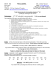### Math Accuplacer Sample Questions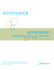### 9th Grade | Unit 1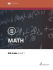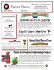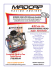### History of Solving Polynomial Equations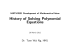### 9.1.1 Simplify Rational Expressions (Algebraic Fractions) Definition rational expression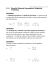### Interpreting Algebraic Expressions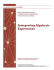### Lipizzaner Stud piber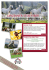### Mathematics Placement Review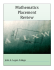### magnificent europe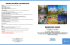### Algebraic Expressions - glencoe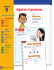### RATE CARD - Montreal Gazette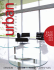### Cheesecake Petit Fours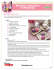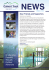### Unit 4 - Amazon Web Services### Guided Help: A Revolution for Software Help and Support?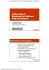### Overcoming Migraine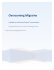### 8 Curriculum Overviews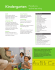### The Saga of Pandora Zwieback #0